Documentation

802.11ax PHY-Focused System-Level Simulation

This example shows how to perform a PHY-focused system-level simulation for IEEE® 802.11ax™. Part (A) validates the simulation scenario, radio characteristics, and large-scale fading model by comparing against published calibration results. Part (B) estimates the packet error rate of the 802.11ax network by simulating individual links between active nodes under a basic clear channel assessment scheme.

Introduction

In this example the performance of an 802.11ax [ 1 ] network in a residential apartment block is evaluated using a PHY-focused system-level simulation.

The residential apartment block simulation scenario is specified in [ 2 ]. This consists of a building with five floors, and twenty 10m x 10m x 3m apartments per floor. Each apartment has an access point (AP) and one or more stations (STAs) placed in random xy-locations, a process referred to as 'dropping' nodes. This creates a basic service set (BSS) per apartment which is randomly assigned one of three channels. The simulation scenario specifies a large-scale path loss model based on the distance between nodes, and the number of walls and floors traversed.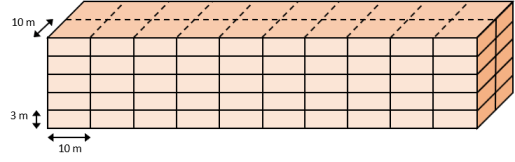The TGax evaluation methodology [ 3 ] for 'PHY System Simulation' is followed for this example:

1. APs and STAs are randomly 'dropped' within the scenario.

2. For each pair of nodes, the large-scale path loss is calculated.

3. One or more 'transmission events' is performed. Each transmission event consists of selecting active APs and STAs based on channel access rules and determining the performance of each link.

This example consists of two parts:

In part (A), the 'calibration' stage, the signal-to-interference-plus-noise ratio (SINR) is calculated for multiple 'drops', assuming downlink interfering transmissions. SINR captures long-term radio characteristics. The cumulative distribution function (CDF) of the SINR is compared with published results from the TGax Task Group [ 4 ].

In part (B), the 'PHY system-level simulation' stage, for each transmission event the PHY layer is modeled for individual links. A basic clear channel assessment (CCA) scheme is used to control which APs are active. Waveforms for the signal of interest and interference, impaired by fading channel models, are generated and combined. The resultant packets are processed by a receiver to recover the packet of interest. The average packet error rate for the network is calculated.

The two parts of this example can be disabled using the parameters calibrate and systemLevelSimulation. A figure displaying the simulation scenarios, nodes, active links, and interference is displayed when showScenarioPlot is true.

calibrate = true;             % To execute Part A calibration test
systemLevelSimulation = true; % To execute Part B system-level simulation
showScenarioPlot = true;      % To show dynamic simulation plotting updates

Simulation Parameters

The major simulation parameters are defined as either belonging to Physical Layer (PHY), Medium Access Control Layer (MAC), scenario, or simulation. In this example the PHY and MAC parameters are assumed to be the same for all nodes.

PHYParameters = struct;
PHYParameters.TxPower = 20;      % Transmitter power in dBm
PHYParameters.TxGain = 0;        % Transmitter antenna gain in dBi
PHYParameters.RxGain = -2;       % Receiver antenna gain in dBi
PHYParameters.NoiseFigure = 7;   % Receiver noise figure in dB
PHYParameters.NumTxAntennas = 1; % Number of transmitter antennas
PHYParameters.NumRxAntennas = 1; % Number of receiver antennas
PHYParameters.ChannelBandwidth = 'CBW80'; % Bandwidth of system
PHYParameters.TransmitterFrequency = 5e9; % Transmitter frequency in Hz

MACParameters = struct;
MACParameters.NumChannels = 3; % Number of non-overlapping channels
MACParameters.CCALevel = -70;  % Transmission threshold in CCA algorithm (dBm)

The scenario parameters define the size and layout of the residential building as per .

% Number of Rooms in [x,y,z] directions
ScenarioParameters = struct;
ScenarioParameters.BuildingLayout = [10 2 5];

% Size of each room in meters [x,y,z]
ScenarioParameters.RoomSize = [10 10 3];

% Number of receivers per room. Note that only one receiver (STA) can be
% active at any given time.
ScenarioParameters.NumRxPerRoom = 1;

The NumDrops and NumTxEventsPerDrop parameters control the length of the simulation. For this example, these parameters are configured for a short simulation, but for meaningful results these should be increased.

A 'drop' randomly places transmitters and receivers within the scenario and selects the channel for a BSS. A 'transmission' event randomly selects transmitters and receivers for transmissions according to basic channel access rules.

SimParameters = struct;
SimParameters.NumDrops = 3;
SimParameters.NumTxEventsPerDrop = 2;

Generate Transmitter Sites

Before the main body of the simulation, the transmitter site objects txsite are generated and assigned room names of the form 'Room#' for ease of reference. One transmitter (AP) per room is assumed. Each transmitter is assumed to be isotropic.

% Total number of transmitters, assuming one transmitter (tx) per room
numTx = prod(ScenarioParameters.BuildingLayout);

% Create transmitter sites with and isotropic antenna element
roomNames = strings(1,numTx);
for siteInd = 1:numTx
roomNames(siteInd) = "Room " + siteInd;
end
txs = txsite('Name',roomNames,...
'TransmitterFrequency',PHYParameters.TransmitterFrequency, ...
'TransmitterPower',10.^((PHYParameters.TxPower+PHYParameters.TxGain-30)/10),...
'Antenna','isotropic');

The receive site objects rxsite are generated and assigned names of the form 'Room#-STA#' for ease of reference. The Scenario parameter NumRxPerRoom is used to define how many receivers (STAs) are present in each room. Each receiver is assumed to be isotropic.

% Total number of receivers, assuming one transmitter (tx) per room
numRx = numTx*ScenarioParameters.NumRxPerRoom;

% Create receiver sites
roomNames = strings(1,numRx);
for siteInd = 1:numRx
roomNames(siteInd) = "Room " + (mod(siteInd-1,numTx)+1) + "-" + ceil(siteInd/numTx);
end
rxs = rxsite('Name',roomNames,'Antenna','isotropic');

% Receiver noise power in dBm
T = 290; % Temperature (Kelvin)
k = physconst('Boltzmann'); % Boltzmann constant
% Sample rate (Hz)
fs = wlanSampleRate(wlanHESUConfig('ChannelBandwidth',PHYParameters.ChannelBandwidth));
rxNoisePower = 10*log10(k*T*fs)+30+PHYParameters.NoiseFigure;

Part A - Align Long-Term Radio Characteristics

In this section, the simulation scenario, radio characteristics, and large-scale fading model are verified by performing the TGax Evaluation Methodology Box 1 Test 2 Downlink Only calibration test [ 3 ]. This test calculates the SINR at all receivers (STAs) assuming all transmitters (APs) are active. Multiple drops of transmitters and receivers are performed as part of the simulation. One active receiver is selected per drop.

The SINR for each receiver is calculated and aggregated over all drops simulated to generate a CDF curve. This curve is compared with the calibration results provided in [ 4 ].

A plot showing the node positions, active links, and interfering links is generated per drop. Individual channels can be hidden and shown in the plot by clicking the corresponding legend entry.

seed = rng(6); % Seed random number generator and store state

if showScenarioPlot
hGrid = tgaxBuildResidentialGrid(ScenarioParameters.RoomSize,ScenarioParameters.BuildingLayout, ...
numTx,numRx,MACParameters.NumChannels);
end

if calibrate

fprintf('Running calibration ...\n');

% Pre-allocate output
output = struct;
output.sinr = zeros(SimParameters.NumDrops,numTx); % for storing SINR values
for drop = 1:SimParameters.NumDrops
% Drop receivers in each room
[association,txChannels,rxChannels,txPositions,rxPositions] = tgaxDropNodes( ...
txs,rxs,ScenarioParameters,MACParameters.NumChannels);

% All transmitters active
activeTx = true(numTx,1);

% Only pick one receiver per Room
rxAlloc = randi([1 ScenarioParameters.NumRxPerRoom],numTx,1);
activeRx = reshape(rxAlloc==1:ScenarioParameters.NumRxPerRoom,[],1);

% Generate propagation model
propModel = TGaxResidential('roomSize',ScenarioParameters.RoomSize);

% Get the index of the transmitter for each receiver
tnum = repmat((1:numTx),1,numRx/numTx);

% SINR calculation - loop over each non-overlapping channel
numChannels = numel(unique(txChannels));
for k = 1:numChannels
% Use kth non-overlapping channel
tind = txChannels == k;
rind = false(size(activeRx));
rind(activeRx) = rxChannels(activeRx) == k;
% Get the index of the transmitter of interest for each active receiver
tsigind = tnum(rind);

% Calculate SNR
output.sinr(drop,tind) = sinr(rxs(rind),txs(tind),...
'PropagationModel',propModel,...
'SignalSource',txs(tsigind), ...
'Map','none');
end

% Plot nodes and links
if showScenarioPlot % update plot data
sprintf('Box 1 Test 2 "downlink only" calibration, drop #%d/%d',drop,SimParameters.NumDrops));
end
end

% Plot the CDF of SINR and compare with calibration curves

fprintf('Calibration complete \n')

end
Running calibration ...
Calibration complete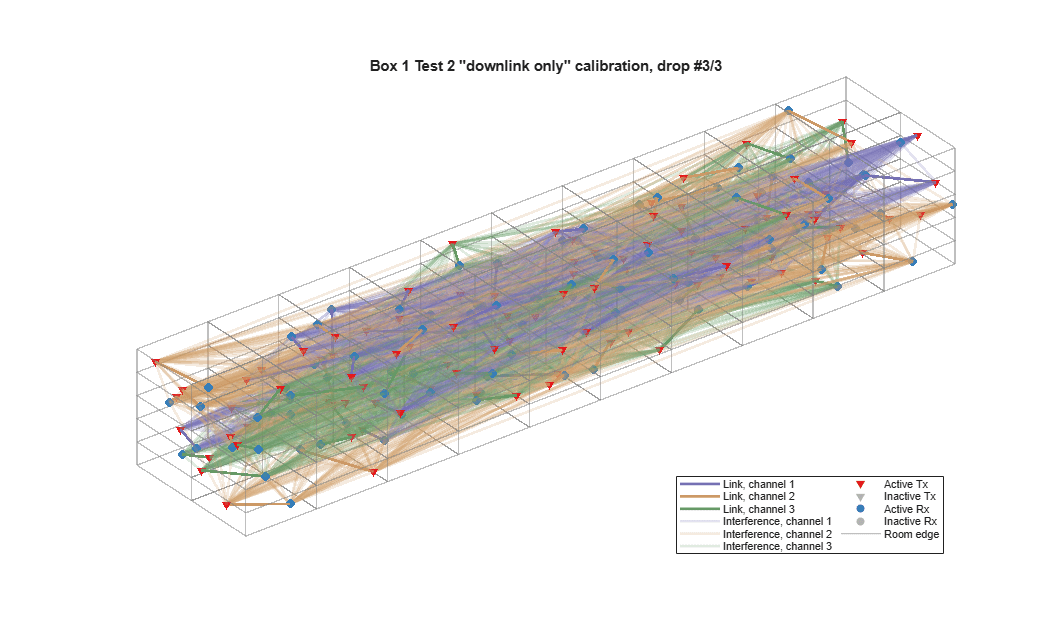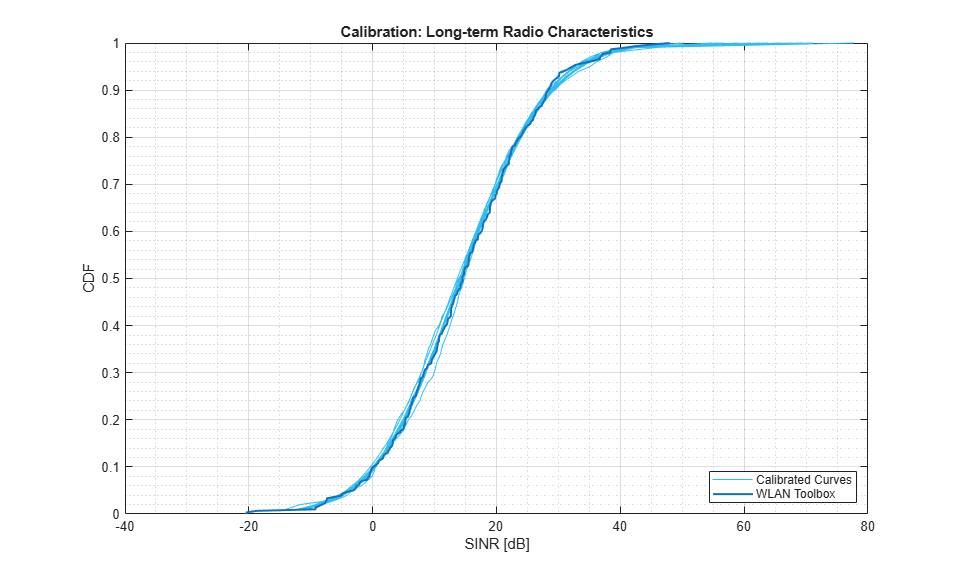This example simulates a small number of drops. Therefore, for a more meaningful comparison the number of drops simulated should be increased. The calibration result for 100 drops is shown below: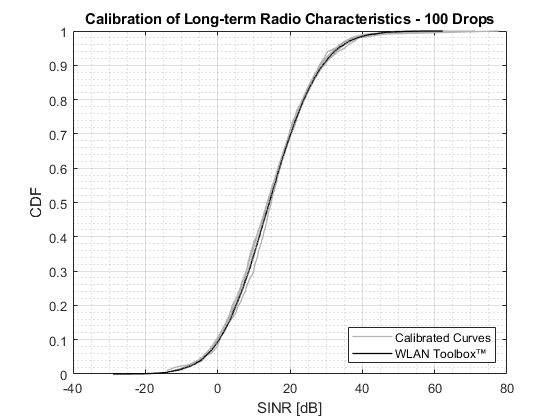Part B - PHY-focused System-Level Simulation

In this section, the scenario and path-loss model calibrated in part A are used to perform a PHY-focused system-level simulation and determine the packet error rate for the network. This simulation is described as PHY-focused as the PHY is not abstracted and the MAC is simplified. Each active link is modeled using baseband transmitter and receiver processing. A very simple MAC assumes at each transmission event all transmitters (APs) wish to transmit, and one receiver (STA) per BSS is the recipient. A simple CCA algorithm is used to control channel access between transmitters as specified in Figure 4 of [ 3 ]. The CCA algorithm enables random transmitters if the signal power received from transmitters that have already been activated does not exceed the CCA threshold, MACParameters.CCAThreshold.

In this example the transmission and channel parameters are assumed to be the same for all nodes. The transmission configuration for all packets is one space-time stream, no space-time block coding and 16-QAM rate-1/2 (MCS 3).

if systemLevelSimulation

% Pre-allocate outputs
output.numPkts = zeros(numRx,1);
output.numPktErrors = zeros(numRx,1);
output.sinrMeas = nan(numRx,SimParameters.NumTxEventsPerDrop,SimParameters.NumDrops);
output.sinrEst = nan(numRx,SimParameters.NumTxEventsPerDrop,SimParameters.NumDrops);
output.pktErrorRate = 0;

% For each possible transmitter create a waveform configuration. In this
% example the link and radio parameters are the same for all nodes.
cfgHEBase = wlanHESUConfig;
cfgHEBase.ChannelBandwidth = PHYParameters.ChannelBandwidth; % Channel bandwidth
cfgHEBase.NumTransmitAntennas = PHYParameters.NumTxAntennas; % Number of transmit antennas
cfgHEBase.SpatialMapping = 'Fourier';  % Spatial mapping matrix
cfgHEBase.NumSpaceTimeStreams = 1;     % Number of space-time streams
cfgHEBase.GuardInterval = 0.8;         % Guard interval duration
cfgHEBase.HELTFType = 4;               % HE-LTF compression mode
cfgHEBase.APEPLength = 1e3;            % Payload length in bytes
cfgHEBase.ChannelCoding = 'LDPC';      % Channel coding
cfgHEBase.MCS = 3;                     % Modulation and coding scheme
cfgHE = cell(numTx,1);
for txidx = 1:numTx
cfgHE{txidx} = cfgHEBase;
end

fprintf('Running System Level Simulation ...\n')
for drop = 1:SimParameters.NumDrops
fprintf('  Running drop #%d/%d ...\n',drop,SimParameters.NumDrops);

% Drop receivers in each room
[association,txChannels,rxChannels,txPositions,rxPositions] = tgaxDropNodes( ...
txs,rxs,ScenarioParameters,MACParameters.NumChannels);

% Generate propagation model
propModel = TGaxResidential('roomSize',ScenarioParameters.RoomSize);

% Calculate signal strength for all links
signalStrength = sigstrength(rxs,txs,propModel,'Type','power',...
'ReceiverGain',PHYParameters.RxGain,'Map','none'); % all signal strengths in dBm

% Threshold signals below noise level to reduce simulation time
signalStrength(signalStrength < rxNoisePower) = -Inf;

% Threshold signals that are not on same non-overlapping channel
signalStrength(~(txChannels == rxChannels')) = -Inf;

% simulate and get the linear indices
nonnegligibleMask = signalStrength > -Inf;

% Reset the non-negligible channels to create a new realization for the
% current drop

% For each possible active link in a drop create a channel
% configuration. In this example the link and radio parameters are the
% same for all nodes.
tgaxChan = cell(numel(nonnegligibleIdx),1);
for i = 1:numel(nonnegligibleIdx)
% Index of transmitter for a given link
txIdx = mod(nonnegligibleIdx(i)-1,numTx)+1;
% Channel configuration. The channel realization for each link is
% different as the global random stream is used.
tgaxChanBase = wlanTGaxChannel;
tgaxChanBase.DelayProfile = 'Model-D';
tgaxChanBase.NumTransmitAntennas = cfgHE{txIdx}.NumTransmitAntennas;
tgaxChanBase.TransmitReceiveDistance = 10; % Distance in meters for NLOS
tgaxChanBase.ChannelBandwidth = cfgHE{txIdx}.ChannelBandwidth;
tgaxChanBase.EnvironmentalSpeed = 0; % m/s, stationary
tgaxChanBase.SampleRate = fs;
tgaxChanBase.NormalizeChannelOutputs = false;

% Store in cell array and reset the channel to generate a new
% response
tgaxChan{i} = tgaxChanBase;
reset(tgaxChan{i});
end

for txevent = 1:SimParameters.NumTxEventsPerDrop
fprintf('    Running transmission event #%d/%d ...\n',txevent,SimParameters.NumTxEventsPerDrop);

% Determine active transmitters and receivers with Clear Channel Assessment
[activeTx,activeRx] = tgaxCCA(signalStrength,MACParameters.CCALevel);

% Plot scenario and links
if showScenarioPlot
sprintf('PHY System-Level Simulation, Drop #%d/%d, Transmission Event #%d/%d', ...
drop,SimParameters.NumDrops,txevent,SimParameters.NumTxEventsPerDrop));
end

% Extract elements for active links using activeTx and activeRx
cfgHEActive = cfgHE(activeTx);
associationActive = association(activeTx,activeRx);
signalStrengthActive = signalStrength(activeTx,activeRx);

% Create array containing active channels
tgaxChanActive = cell(size(associationActive));
matchIdx = nonnegligibleIdx==find(activeTx&activeRx');

% Generate a waveform for each non-negligible active link and
% combine waveforms for each receiver
[rxWavs,txPSDUActive,signalPower,interfPower] = tgaxGenerateRxWaveforms( ...

% Run PHY link simulation for each link and determine if the packet
% has been decoded successfully. The estimated interference power
% is passed to the receiver in the place of an interference power
% measurement algorithm.
numActiveRxs = sum(activeRx);
pktError = false(numActiveRxs,1);
sinrMeas = nan(numActiveRxs,1);
for rxIdx = 1:numActiveRxs
[pktError(rxIdx),sinrMeas(rxIdx)] = tgaxModelPHYLink( ...
rxWavs{rxIdx},cfgHEActive{rxIdx},rxNoisePower,interfPower(rxIdx),txPSDUActive{rxIdx});
end

% Store output for active receivers
output.numPktErrors(activeRx) = output.numPktErrors(activeRx)+pktError;
output.numPkts(activeRx) = output.numPkts(activeRx) + 1;
output.sinrMeas(activeRx,txevent,drop) = sinrMeas;

% Calculate the expected SINR at each receiver
sinrEst = 10*log10(signalPower./(interfPower+10^((rxNoisePower-30)/10)));
output.sinrEst(activeRx,txevent,drop) = sinrEst;
end
end

% Calculate average packet error rate
output.pktErrorRate = sum(output.numPktErrors)/sum(output.numPkts);

disp('Simulation complete')
disp(['Average packet error rate for transmitters: ' num2str(output.pktErrorRate)]);

end

rng(seed); % Restore random state
Running System Level Simulation ...
Running drop #1/3 ...
Running transmission event #1/2 ...
Running transmission event #2/2 ...
Running drop #2/3 ...
Running transmission event #1/2 ...
Running transmission event #2/2 ...
Running drop #3/3 ...
Running transmission event #1/2 ...
Running transmission event #2/2 ...
Simulation complete
Average packet error rate for transmitters: 0.054152Further Exploration

The PHY-focused system-level simulation demonstrated in this example can be used to explore the impact of PHY-level parameters on system performance. For example, the plot below shows the network average packet error rate for different values of CCA threshold for 50 drops and 2 transmission events per drop.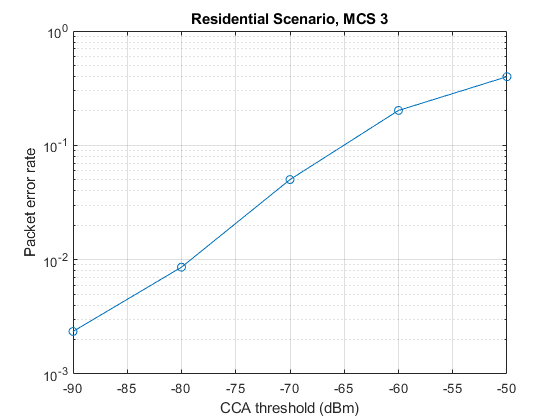Appendix

This example uses the following helper functions:

Selected Bibliography

1. IEEE P802.11ax™/D3.1 Draft Standard for Information technology - Telecommunications and information exchange between systems - Local and metropolitan area networks - Specific requirements - Part 11: Wireless LAN Medium Access Control (MAC) and Physical Layer (PHY) Specifications - Amendment 6: Enhancements for High Efficiency WLAN.

2. IEEE 802.11-14/0980r16 - TGax Simulation Scenarios.

3. IEEE 802.11-14/0571r12 - 11ax Evaluation Methodology.

4. IEEE 802.11-14/0800r30 - Box 1 and Box 2 Calibration Results.

WLAN Toolbox DocumentationGet trial now